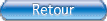let it beThe BeatlesLet it be

Song :
G
Intro :
C   G   Am    F
C   G   F/C   Am/C   Dm7   C

C              G
When I find myself in times of trouble,
Am                   F
Mother Mary comes to me
C                   G            F/C    Am/C   Dm7   C
Speaking words of wisdom, let it be
C             G
And in my hour of darkness,
Am              F
She is standing right in front of me
C                 G             F/C    Am/C   Dm7   C
Speaking words of wisdom, Let it be

Chorus :
Am          G         F          C
Let it be, let it be, let it be, let it be
C                G               F/C    Am/C   Dm7   C
Whisper words of wisdom, let it be

C              G
And when the broken hearted people,
Am            F
Living in the world agree
C                 G              F/C    Am/C   Dm7   C
There will be an answer, let it be
C                 G
But though they may be parted,
Am                F
There is still a chance that they may see
C                G              F/C    Am/C   Dm7   C
There will be an answer, let it be

Chorus :
Am           G         F          C
Let it be, let it be, let it be, let it be
C                G             F/C    Am/C   Dm7   C
There will be an answer, let it be
Am         G          F          C
Let it be, let it be, let it be, let it be
C                G              F/C    Am/C   Dm7   C
Whisper words of wisdom, let it be

Bridge :
F/C    Am/C   Dm7   C     Bb  Am   G    F    C
Solo :
C   G   Am   F   C   G   F/C   Am/C   Dm7   C
C   G   Am   F   C   G   F/C   Am/C   Dm7   C

Chorus :
Am          G          F          C
Let it be, let it be, let it be, let it be
C                 G             F/C    Am/C   Dm7   C
Whisper words of wisdom, let it be

C                G
And when the night is cloudy,
Am                 F
There is still a light that shines on me
C               G              F/C    Am/C   Dm7   C
Shine on till tomorrow, let it be
C                G
I wake up to the sound of music,
Am            F
Mother Mary comes to me
C                     G         F/C    Am/C   Dm7   C
Speaking words of wisdom, let it be

Chorus :
Am          G           F         C
Let it be, let it be, let it be, let it be
C                G              F/C   Am/C   Dm7   C
There will be an answer, let it be
Am         G           F         C
Let it be, let it be, let it be, let it be
C                G              F/C   Am/C   Dm7   C
Whisper words of wisdom, let it be

Outro :
F/C   Am/C   Dm7   C   Bb   Am   G   F/C   C